# 15.10 - How the AMP Software Collects Statistics - Teradata Database

## Teradata Database SQL Request and Transaction Processing

Product
Release Number
15.10
Content Type
Programming Reference
User Guide
Publication ID
B035-1142-151K
Language
English (United States)

When you collect statistics and demographics, the AMP software creates statistics rows in DBC.StatsTbl that stores various statistics and demographics that summarize the table columns specified by the COLLECT STATISTICS statement. These rows come in four basic types.

• Regular statistics rows (one or more per AMP)
• Null rows (zero or one per AMP)
• All‑null rows (zero or one per AMP)
• Average rows per value (one per AMP)
• Teradata Database uses a different method to collect statistics on columns that have a geospatial data type. For information about how the system collects statistics on geospatial columns, see “Collecting Row Statistics and Demographics for Geospatial Columns and Indexes” on page 157, “GeoGrid Population Statistics” on page 158, and “Hilbert R‑Tree Summary Statistics” on page 160.

The information taken from these rows is used to populate the statistical interval histograms used by the Optimizer to make its initial cardinality estimates (see “Interval Histograms” on page 162).

The following statistics are global per histogram, so are reported only once.

• Number of null rows
• Number of all‑null rows
• Overall average of the average number of rows per value per AMP
• You can report these statistics using a HELP STATISTICS request (see “HELP STATISTICS” in SQL Data Definition Language for more information about the HELP STATISTICS statement). Note that HELP STATISTICS only reports summary statistics. To report detailed statistics, you must submit a SHOW STATISTICS request.

Null and all‑null statistics provide the following cardinality information.

 Statistic Definition NumNulls Number of rows in the column set for which one or more of the columns are null. NumAllNulls Number of rows in a composite column set for which all of the columns are null.

Teradata Database only collects the NumAllNulls statistic for composite column sets when all of the columns in the composite set are null. In other words, the only time it is possible to collect a NumAllNulls value is when you collect multicolumn statistics or collect statistics on a composite index, and none of the columns in that set have a value.

Consider the following example of what is meant by null and all‑null rows. Suppose you create a 3‑column USI for table t_ex on columns b, c, and d. When you collect statistics on the USI, one row is found in which all 3 columns making up the USI are null. This would be reported as an all‑nulls instance in the statistics. This example is degenerate because there could never be more than one row having a USI with all null columns in a table, but it is useful as an example, even though it does not generalize.

The columns for this row would look something like the following, where nulls are represented by the QUESTION MARK character.

 t_ex a b c d e f PI USI 355 ? ? ? 25000.00 375000.00

This contrasts with the case where statistics have been collected on a composite column set, and one or more, but not necessarily all, of the columns on which those statistics have been collected for a given row are null.

Assume the same table and index definitions as before. When you collect statistics on the USI, any row found where one or two, but not all, of the three columns making up the USI are null would be reported as an occurrence of a null column in the statistics.

All of the following rows in t_ex would be reported as null fields, because all have at least one null in one of the USI columns, while only the first row would be reported for the all‑null fields statistic.

 t_ex a b c d e f PI USI 355 ? ? ? 25000.00 375000.00 ←   all‑null fields (green) 685 ? 155 ? 45000.00 495000.00 ←   null fields (orange) 900 325 ? ? 32000.00 400000.00 275 ? ? 760 55000.00 575000.00 597 ? 254 893 10000.00 150000.00 322 679 ? 204 75000.00 650000.00 781 891 357 ? 32000.00 400000.00

All‑nulls describes the case where statistics have been collected on a composite column set, and all the columns on which those statistics have been collected for a given row are null. With this information, the Optimizer can more accurately estimate the true number of unique values in a set, thus enabling more accurate join costing.

For example, suppose you have table t1 with columns x1 and y1, and values for x1 and y1.

Again, the all nulls composite fields are shaded orange and the partly null composite fields are shaded green.

If you could collect only the NumNulls statistic, then when you collected statistics on composite columns, and one or more of the columns was null, the row would be counted as a null row. This is not an issue for single‑table cardinality estimation because a comparison against a null is always evaluated as FALSE and is treated as an unmatched row.

For example, if you could collect only the NumNulls statistic, the histogram on (x1, y1) would indicate that the number of nulls is 4, the number of unique values is 2, and the total number of rows is 5. If columns x and y are used as join columns, the Optimizer would evaluate the join costing by incorrectly assuming there are only 2 unique values in the data when there are actually 4. This can cause problems in scenarios such as redistribution costs, skew detection, and the like.

To circumvent this problem, Teradata Database computes the true number of distinct partial nulls and saves them in the histogram as NumPNulls.

Teradata Database uses the following equation to calculate the true distinct values for partial nulls.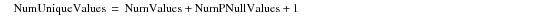where:

 Equation element … Specifies … NumUniqueValues the number of true distinct values for partially null rows. NumValues the number of non‑distinct values for partially null rows. NumPNullValues the number of true distinct values among the partially null rows. 1 an adjustment for the estimation error when the cardinality of all nulls > 0.

To make this computation, Teradata Database injects a marker and uses the following aggregation query to collect statistics on (x1,y1). For the following request, the fieldID for null_marker is set to 2 and the fieldID for cnt is set to 3.

`     SELECT CASE `
`                WHEN x1 IS NULL `
`                AND  y1 IS NULL `
`                THEN 1 `
`                ELSE IF x1 IS NULL `
`                OR `
`                        y1 IS NULL `
`                THEN 2 `
`            ELSE 0 `
`            END AS null_marker ,x1, y1, COUNT(*) AS cnt`
`     FROM t_coll_stats`
`     GROUP BY 1, 2;`

To collect sampled statistics, Teradata Database generates a retrieve request to do the sampling. It then passes the sampled rows to the subsequent aggregation step. The retrieve request can use TOP n operations to retrieve the rows if the table is hash distributed and either hard ordered or a NoPI table. For PPI tables that are sorted on their RowKey value, Teradata Database can generate a true sample step.

The aggregation step builds a detailed row with the partition number and the cardinality for queries such as the following.

`     SELECT PARTITION, COUNT(*) `
`     FROM t_coll_stats; `

You can collect statistics on deterministic UDFs, but not on nondeterministic UDFs.

Consider the following deterministic UDF.

`     CREATE FUNCTION months_between(date1 TIMESTAMP, date2 TIMESTAMP) `
`     RETURNS FLOAT`
`     LANGUAGE C `
`     NO SQL`
`     DETERMINISTIC`
`     SPECIFIC months_between_tt`
`     EXTERNAL NAME 'CS!months_between_tt!\$PGMPATH\$/months_between_tt.c'`
`     PARAMETER STYLE SQL;`

The following statement collects statistics on UDF months_between().

`     COLLECT STATISTICS `
`     COLUMN months_between(BEGIN(policy_duration),`
`                           END(policy_duration)) AS                            Stats_MthsBetweenBegAndEnd`
`     ON policy_types;`

The crucial issue for UDF statistics is not collecting them, but dropping them. If you drop a deterministic UDF after you have collected statistics on it, those statistics become unusable. Because UDFs do not belong to any table or column, it is very expensive to drop all the statistics collected on them. Instead, Teradata Database uses the following lazy approach to dropping statistics that have been collected on deterministic UDFs.

• When Teradata Database loads statistics header information from the DBC.StatsTbl for a table to handle a user query or another statistics‑related statement, the system detects nonvalid expressions at the time the query is resolved.
• For statistics collected on an unresolvable expression, the system updates the validStats field to FALSE for the corresponding statistics in DBC.StatsTbl.

• When retrieving histograms, Teradata Database only retrieves those for valid statistics. This minimizes the overhead of parsing nonvalid statistics during query processing time.
• The SHOW STATISTICS and HELP STATISTICS statements report only valid statistics.
• A DROP STATISTICS request on a table forces Teradata Database to drop all statistics, both valid and nonvalid.
• A SHOW STATISTICS … COLUMN request returns a warning message if it is made on nonvalid statistics.
• A DROP STATISTICS …COLUMN request returns a warning message if it is made on nonvalid statistics.
• Recollecting statistics on a UDF revalidates the statistics if the UDF exists at the time the statistics are recollected.
• You can query the DBC.StatsV system view to determine nonvalid statistics by checking the validStats column
• You cannot drop individual nonvalid statistics.

To drop nonvalid statistics, you must first drop all statistics on the table and then recollect valid statistics.

• If you drop a UDF and then recreate it to return a different type, Teradata Database ignores the existing statistics for that function during optimization.
• The average AMP rows per value statistic is an exact system‑wide average of the cardinality per value for each individual AMP over the number of rows per value for a NUSI column set on which statistics have been collected. This statistic is computed only for NUSI columns and is used for nested join costing.

All AMPs compute the AMP-level rows per value on base tables and index tables to determine the row cardinality and number of values respectively. Teradata Database calculates the AMP‑level rows per value statistic as follows.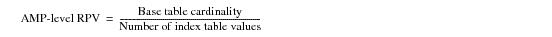where:

 Equation element … Specifies the … AMP‑level RPV AMP‑level rows per value.

All of the AMPs send their local amp-level rows per value to the driving AMP, which is determined based on the hash of its object ID) of the collect statistics update step. Once the amp-level RPV is received, the collect statistics logic calculates the Average AMP Rows Per Value by taking the average of amp‑level RPV as follows.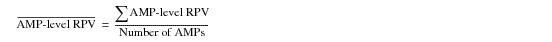where:

 Equation element … Specifies the …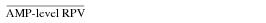Average AMP‑level rows per value.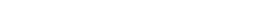Sum of all AMP‑level rows per value.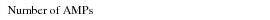Number of AMPs in the system.

The following EXPLAIN text reports the steps Teradata Database takes to build a histogram and collect statistics for the first time on column x1 from table t1 with embedded comments to clarify exactly what happens at critical steps in the process.

`     EXPLAIN COLLECT STATISTICS COLUMN x1 ON t1;`
` `
`Explanation`
`---------------------------------------------------------------------------`
`  1) First, we lock QSTATS.t1 for access.`
`  2) Next, we do an all-AMPs SUM step to aggregate from DF2.t1 by way of`
`     an all-rows scan with no residual conditions,`
`     grouping by field1 ( RK.t1.x1).  Aggregate Intermediate Results`
`     are computed locally, then placed in Spool 9.  The size of Spool 9`
`     is estimated with low confidence to be 2 rows (44 bytes).  The`
`     estimated time for this step is 0.03 seconds.`
`  3) We do an all-AMPs RETRIEVE step from Spool 9 (Last Use) by way of`
`     an all-rows scan into Spool 5 (all_amps), which is built locally`
`     on the AMPs.  The size of Spool 5 is estimated with low confidence`
`     to be 2 rows (52 bytes).  The estimated time for this step is 0.04`
`     seconds.`
`  4) Then we save the UPDATED STATISTICS from Spool 5 (Last Use) into`
`     Spool 3, which is built locally on the AMP derived from`
`     DBC.StatsTbl by way of the primary index. /*Hash of t1*/`
`  5) We lock DBC.StatsTbl for write on a RowHash.`
`   /*Raise a retryable error if the generated stats id is already used */`
`   /*by some other collect stats on the same table.*/`
`   /*The error step can also be done by USI on (TableId, StatsId)*/`
`  6) We do a single-AMP ABORT test from DBC.StatsTbl by way of the`
`     primary index "DBC.StatsTbl.TableId = <t1 Id> with a residual `
`     condition of ("DBC.StatsTbl.StatsId = <generated stats id>").`
`   /*Insert the histogram row with generated stats id*/`
`  7) We do a single-AMP MERGE into DBC.StatsTbl from Spool 3 (Last Use).`
`     The size is estimated with low confidence to be 1 row.  The`
`     estimated time for this step is 0.31 seconds.`
`    /*Update or insert the master record with the updated table level demographics*/`
`  8) We do a single-AMP UPDATE from Spool 3 by way of the primary`
`     index "DBC.StatsTbl.TableId = <t1 Id>" with a residual condition of `
`     DBC.StatsTbl.StatsId = 0. The size is`
`     estimated with low confidence to be 1 row.  The estimated time for`
`     this step is 0.02 seconds.  If the row cannot be found, then we do`
`     an INSERT into DBC.StatsTbl.`
`  9) We spoil the parser's dictionary cache for the table.`
`  10) Finally, we send out an END TRANSACTION step to all AMPs involved`
`     in processing the request.`
`  -> No rows are returned to the user as the result of statement 1.`
` `

Note the following additional aspects of this EXPLAIN text.

• In step 7, Teradata Database inserts the histogram row with the generated StatsId.
• In step 8, Teradata Database updates the master record with new table‑level demographics with a fixed StatsId of 0.
• Note that master records always have a fixed StatsId of 0.
• The following EXPLAIN text reports the steps Teradata Database takes to recollect statistics on column x1 from table t1 with embedded comments to clarify exactly what happens at critical steps in the process.

`     EXPLAIN COLLECT STATISTICS COLUMN x1 ON t1;`
` `
`Explanation`
`---------------------------------------------------------------------------`
`  1) First, we lock QSTATS.t1 for access.`
`  2) Next, we do an all-AMPs SUM step to aggregate from RK.t1 by way of`
`     an all-rows scan with no residual conditions,`
`     grouping by field1 ( RK.t1.x1).  Aggregate Intermediate Results`
`     are computed locally, then placed in Spool 9.  The size of Spool 9`
`     is estimated with low confidence to be 2 rows (44 bytes).  The`
`     estimated time for this step is 0.03 seconds.`
`  3) We do an all-AMPs RETRIEVE step from Spool 9 (Last Use) by way of`
`     an all-rows scan into Spool 5 (all_amps), which is built locally`
`     on the AMPs.  The size of Spool 5 is estimated with low confidence`
`     to be 2 rows (52 bytes).  The estimated time for this step is 0.04`
`     seconds.`
`  4) Then we save the UPDATED STATISTICS from Spool 1 (Last Use) into`
`     Spool 3, which is built locally on the AMP derived from`
`     DBC.StatsTbl by way of the primary index.`
`  5) We lock DBC.StatsTbl for write on a RowHash. /*Hash of (t1)*/`
`  6) We do a single-AMP UPDATE from Spool 3 by way of the primary`
`     index "DBC.StatsTbl.TableId = <t1 Id>" with a residual condition of `
`     DBC.StatsTbl.StatsId = <existing stats id>.  The size is`
`     estimated with low confidence to be 1 row.  The estimated time for`
`     this step is 0.02 seconds.  If the row cannot be found, then we do`
`     an INSERT into DBC.StatsTbl.`
`  7) We do a single-AMP UPDATE from Spool 3 by way of the primary`
`     index "DBC.StatsTbl.TableId = <t1 Id>" with a residual condition of `
`     DBC.StatsTbl.StatsId = 0. The size is`
`     estimated with low confidence to be 1 row.  The estimated time for`
`     this step is 0.02 seconds.  If the row cannot be found, then we do`
`     an INSERT into DBC.StatsTbl.`
`  8) We spoil the parser's dictionary cache for the table.`
`  9) Finally, we send out an END TRANSACTION step to all AMPs involved`
`     in processing the request.`
`  -> No rows are returned to the user as the result of statement 1.`
` `

Note the following additional aspects of this EXPLAIN text.

• In step 6, Teradata Database updates the histogram row with the existing StatsId.
• In step 7, Teradata Database updates the master record with new table‑level demographics with a fixed StatsId of 0.
• Master records always have a fixed statistics ID of 0.
• Teradata Database collects statistics on a base table using the following method, which automatically uses all of the access paths that are available for aggregation processing such as covering indexes, join indexes (including aggregate join indexes), and hash indexes to do the SUM step processing.

1 Generate a regular SUM step to aggregate on the statistics columns.

For example, for statistics on (x1, y1) from table t_coll_stats, Teradata Database executes the following request.

`     SELECT x1, y1, COUNT(*) AS cnt `
`     FROM t_coll_stats `
`     GROUP BY 1,2;`

In addition to the aggregation, Teradata Database also captures the source row count, which it uses to determine local and global interval table sizes.

2 Teradata Database takes the input of this global aggregated spool and follows these steps to build the interval histogram.

a Build the interval table.

b Determine the histogram boundaries from the interval table.

c Broadcast the skeleton histogram to all of the AMPs.

d Each AMP reads the aggregated spool and fills its local histogram.

e The system does a final consolidation of the local histograms.

f The consolidated histogram row is ready to be updated to DBC.StatsTbl.

The following table shows how each individual AMP aggregates its regular, null, all‑null, and average AMP rows per value statistics rows for columns that do not have a geospatial data type.

There are one or more regular rows; zero or one null rows; zero or one all-null rows; and one average rows per value row per AMP.

 Row Type Column 1 Column 2 Column 3 Average AMP RPV 0 Local Average RPV 0 All-Null 0 Negative cardinality for rows with all columns null Null Null 0 Cardinality for rows with one or more columns null Null Regular Ynet key Cardinality Value Ynet key Cardinality Value … … … Ynet key Cardinality Value

Note: When the column set is not a NUSI, the value for Average AMP RPV is always reported as 0. The following example illustrates the Average AMP RPV statistic.

First create the table on which statistics are to be collected.

`     CREATE TABLE demo_table (`
`       x INTEGER, `
`       y INTEGER, `
`       z INTEGER)`
`     PRIMARY INDEX(x)`
`     INDEX (y,z);`

Notice the NUSI on columns y and z.

Now populate the table with values. There are no nulls in any of the resulting columns.

`     INSERT INTO demo_table VALUES (1,1,1);`
`     INSERT INTO demo_table VALUES (2,2,1);`
`     INSERT INTO demo_table VALUES (3,2,1);`
`     INSERT INTO demo_table VALUES (4,3,8);`
`     INSERT INTO demo_table VALUES (5,3,8);`
`     INSERT INTO demo_table VALUES (6,3,8);`
`     INSERT INTO demo_table VALUES (7,6,7);`
`     INSERT INTO demo_table VALUES (8,6,7);`
`     INSERT INTO demo_table VALUES (9,6,7);`
`     INSERT INTO demo_table VALUES (10,6,7);`

The following query reports the distribution of these rows across the 2 AMPs in the system, AMP0 and AMP1.

`     SELECT HASHAMP(HASHBUCKET(HASHROW(x))) AS ampno, x, y, z `
`     FROM demo_table `
`     GROUP BY 1,2,3,4 `
`     ORDER BY 1;`
` `
`           ampno            x            y            z`
`     -----------  -----------  -----------  -----------`
`               0            3            2            1`
`               0            9            6            7`
`               0            5            3            8`
`               0            2            2            1`
`               0            6            3            8`
`               1            8            6            7`
`               1           10            6            7`
`               1            4            3            8`
`               1            7            6            7`
`               1            1            1            1`

Now count the rows according to their value distribution in AMP0 and AMP1.

 AMP Number Value of (y,z) Cardinality 0 (2,1) 2 (6,7) 1 (3,8) 2 1 (6,7) 3 (3,8) 1 (1,1) 1

From the distribution, it is possible to calculate the average rows per value for each AMP.

The values for each AMP are: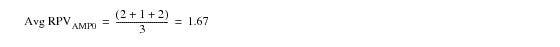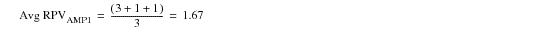At the local aggregation stage of statistics collection, the following statistics rows exist on a two-AMP system according to the hash function.

 AMP Number Column 1 Column 2 Column 3 0 0 1.67 0 Ynet(2,1) 2 (2,1) Ynet(6,7) 1 (6,7) Ynet(3,8) 2 (3,8) 1 0 1.67 0 Ynet(6,7) 3 (6,7) Ynet(3,8) 1 (3,8) Ynet(1,1) 1 (1,1)

In abbreviated tabular form, the average rows per value per AMP are as follows.

 AMP Number Average Rows Per Value 0 1.67 1 1.67

The system‑wide average AMP-local RPV is therefore the average of the two.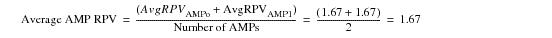For information about how Teradata Database collects statistics and demographics for geospatial columns, refer to the topics in the following list.

• “Collecting Row Statistics and Demographics for Geospatial Columns and Indexes” on page 157
• “GeoGrid Population Statistics” on page 158
• “Hilbert R‑Tree Summary Statistics” on page 160
• Teradata Database uses a different method to collect statistics on columns and NUSIs that have a geospatial data type.

The system uses 2 components when it collects statistics on geospatial columns and NUSIs.

• A GeoGrid histogram that is used for collecting statistics on both geospatial columns and geospatial indexes.
• This component contains column population statistics and demographic data that is stored as part of the interval histogram for a statistics collection in DBC.StatsTbl.

Teradata Database creates a GeoGrid histogram when you first collect statistics on both geospatial columns and geospatial indexes.

• A system‑wide composite Hilbert R‑tree that is used only for NUSIs created on geospatial columns.
• This component contains Minimum Bounding Rectangle (MBR) index data that approximates the worst case summary data about elements such as the following.

• Hilbert R‑tree height
• Number of directory blocks
• Number of directory rows
• Number of leaf blocks
• Number of leaf rows
• Teradata Database creates a Hilbert R‑tree when you first collect statistics on a geospatial index, but not when you first collect statistics on a geospatial column that is not also a NUSI.

Unlike a GeoGrid histogram, the Hilbert R‑tree is stored on top of the Teradata file system, not in DBC.StatsTbl.

Teradata Database collects statistics on geospatial columns in 2 phases.

1 The system selects a centralized point on a master AMP requests each of the AMPs to return an AMP‑local MBR that represents an approximation of the boundary of all the spatial objects stored within the table column on that AMP. Teradata Database then combines the individual AMP-local MBRs into a single MBR that represents the known universe for all spatial objects stored in that column on all AMPs.

This system-wide universe MBR is divided into n equal‑sized grids.

2 The master AMP from phase 2 of the process broadcasts the information about the universe MBR and the grids to all the AMPs.

The AMPs read the rows from their portion of the table and fill in per grid count information. When this process is complete, each AMP has an AMP-local perspective of the statistics on the spatial column. The AMPs then transmit their AMP-local results to the centralized point, where the AMP-local results are combined into a global result that contains the statistics relative to the column across all AMPs. The global results, referred to as a GeoGrid, are then written to DBC.StatsTbl.

Note: 3-dimensional geospatial data uses a 2-dimensional geospatial indexing infrastructure. 3-dimensional data is indexed using only its X and Y coordinates; the Z coordinates are ignored. In cases where the data is stacked vertically or where there is significant vertical overlap, a geospatial index does not provide much performance improvement. See SQL Geospatial Types for more information about geospatial indexes.

Teradata Database stores population statistical data collected on geospatial columns within a variable‑sized structure in DBC.StatsTbl, the size of which depends on the precision required to store the population data.

A COLLECT STATISTICS request captures a snapshot of the system at the time the request was submitted. For geospatial data, which is spatial objects in x-y space, you can think of the statistics snapshot for the population as a rectangle in x-y space bounded by a set of (Xmin, Ymin) and (Xmax, Ymax) coordinates. This rectangle can then be divided into a number of equally‑sized, smaller rectangles referred to as grids.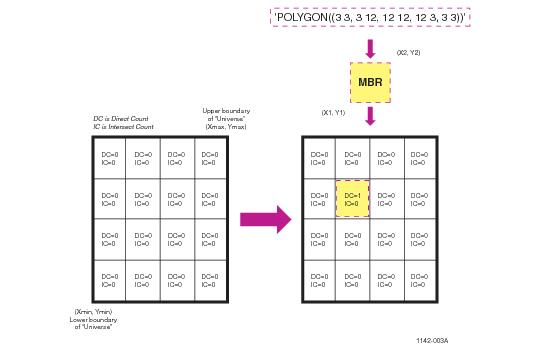Teradata Database maintains 2 statistical counts for each grid.

• Distinct count
• This count is incremented for each MBR that is wholly contained within the grid.

• Intersect count
• This count is incremented whenever one of the following cases occurs.

• A minimum bounding rectangle (MBR) crosses multiple grids that include this grid.
• An MBR touches one or more boundaries of this grid.
• The following graphic indicates how Teradata Database records population data in the GeoGrid for MBRs that intersect multiple grids with a GeoGrid.

The right side of the graphic indicates that the geometric shape of a polygon can be approximated by the MBR having the coordinates of (X1, Y1) and X2, Y2). This MBR spans the 4 grids highlighted by the shaded region in the drawing, so Teradata Database increments the Intersect counts of all 4 grids by 1.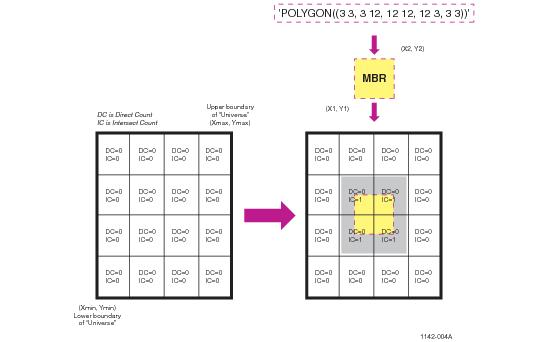The following website contains a wealth of introductory information about using various geospatial methods to search relational database management systems for geospatial data: http://geospatialmethods.org/.

During the same phases used to collect the information used to construct the portion of the GeoGrid structure that contains population statistical and demographic data, Teradata Database also collects and assembles the information it needs to formulate a summary Hilbert R‑tree statistical component.

Each local per-AMP Hilbert R‑tree maintains a series of counts.

• Number of nulls
• Number of directory blocks
• Number of directory rows
• Number of leaf blocks
• Number of leaf rows
• Number of R‑Tree levels
• The system uses these counts to assemble an approximate R‑Tree that models the R‑Tree stored at that AMP location. Each AMP can assemble its own AMP-local model with the following process.

1 Divide the number of directory rows by the number of directory blocks to determine the approximate number of rows per directory block.

2 Divide the number of leaf rows by the number of leaf blocks to determine the approximate number of rows per leaf block.

3 Teradata Database constructs an estimated R‑Tree as a number of levels R‑Tree whose directory blocks and leaf blocks are estimated to contain the number of rows per block as calculated in the previous 2 stages.

The individual AMPs then transmit their AMP-local results to a central staging point that combines the AMP-local results into a global result by determining the highest directory row per block, leaf row per block, and number of tree level values. The resulting R‑Tree approximates the worst-case composite system-wide R‑Tree. Teradata Database then writes the global result, referred to as the composite system-wide R‑Tree statistical component, to DBC.StatsTbl.